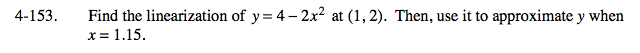### Home > CALC > Chapter 4 > Lesson 4.4.3 > Problem4-153

4-153.Recall that you first investigated local linearization by using your calculator to 'zoom in' on a coordinate point until the curvy function appeared to be linear. You then found the equation of that 'line' using two points. You now have a better strategy.

Find the equation of the tangent line at (1, 2) then evaluate x = 1.15.